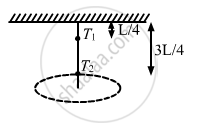Department of Pre-University Education, KarnatakaPUC Karnataka Science Class 11

# A Rod of Length L is Pivoted at One End and is Rotated with a Uniform Angular Velocity in a Horizontal Plane. Let T1 and T2 Be the Tensions at the Points L/4 and 3l/4 Away from the Pivoted Ends. - Physics

#### Question

A rod of length L is pivoted at one end and is rotated with a uniform angular velocity in a horizontal plane. Let T1 and T2 be the tensions at the points L/4 and 3L/4 away from the pivoted ends.

##### Options
• T1 > T2

• T2 > T1

• T1 = T2

• The relation between T1 and T2 depends on whether the rod rotates clockwise or anticlockwise.

#### Solution

T> TLet the angular velocity of the rod be $\omega$ .

Distance of the centre of mass of portion of the rod on the right side of L/4 from the pivoted end :

$r_1 = \frac{L}{4} + \frac{1}{2}\left( \frac{3L}{4} \right) = \frac{5L}{8}$

Mass of the rod on the right side of L/4 from the pivoted end : $\text{m}_1 = \frac{3}{4}\text{M}$

At point L/4, we have :

$T_1 = \text{ m}_1 \omega^2 \text{ r}_1$

$= \frac{3}{4}\text{ M } \omega^2 \frac{5}{8}\text{ L} = \frac{15}{32}\text{ M }\omega^2 \text{ L}$

Distance of the centre of mass of rod on the right side of 3L/4 from the pivoted end :

$\text{r}_1 = \frac{1}{2}\left( \frac{L}{4} \right) + \frac{3L}{4} = \frac{7L}{8}$

Mass of the rod on the right side of L/4 from the pivoted end : $\text{m}_1 = \frac{1}{4}\text{M}$

At point 3L/4, we have :

$\text{T}_2 = \text{m}_2 \omega^2 \text{r}_2$

$= \frac{1}{4}\text{M} \omega^2 \frac{7}{8}\text{L} = \frac{7}{32}\text{M} \omega^2 \text{L}$

∴ T1 > T

Is there an error in this question or solution?

#### APPEARS IN

HC Verma Class 12 Concepts of Physics 1
Chapter 7 Circular Motion
MCQ | Q 14 | Page 113